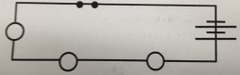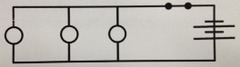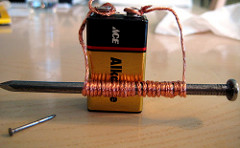# Electricity and Magnetism Study Guide (8th Grade)James Hopper
question

Charge

A physical property resulting from the collection of, or dispersion, of electrons.
question

Static Electricity

A buildup of charges on an object. Generally produced by friction or induction.
question

Charge via Friction

As two objects rub together, electrons from one object are transferred from one to the other.
question

Induction

Description: A negative(ly) charged object – when placed near a neutral object – causes the electrons on another object to be repelled, leaving a positively charged surface because only the electrons can move – the protons are locked in place by the nucleus.
question

Conduction

Description: A negatively charged object (like a plastic ruler) transfers some of its electrons to a neutral object when they touch.
question

Electricity

A form of energy caused by the movement of electrons. The flow of electrons.
question

Direct Current

Direct current (known as DC) is a current that flows through a conductor in one direction only.
question

Direct Current Examples

Battery powered laptops, cellphones or flashlights.
question

Alternating Current

Alternating Current (known as AC) and is a flow of electric charge that regularly reverses directions.
question

Alternating Current Examples

In anything used in your home or on the main power grid from the electric company: TV, vacuum cleaner, microwave.
question

Voltage

The potential difference measured in volts, and the amount of work to be done to move a charge from one point to another. The \”V\” in V=IR
question

Current

A movement of electrical charges around a closed path or circuit, measured in amperes (amps, A), the I in V=IR
question

Resistance

A material’s opposition to the flow of electric current. The R in V=IR
question

V=IR

The relationship between voltage, resistance and current. Voltage is equal to current times resistance (V=IR).
question

It uses less wire and energy so your batteries will last longer.
question

The current is shared, so each resistor gets less energy (i.e, each light bulb will be dimmer) and if one bulb breaks all others will stop working as well.
question

Diagram: Series CircuitWith Battery, Switch, and 3 Resistors
question

The resistors each get the full benefit of the charge (i.e., lights will be brighter), and if one break all others continue to function.
question

They require more energy so your battery won’t last as long, they use more wire as each resistor requires its own path.
question

Diagram: Parallel CircuitWith Battery, Switch, and 3 resistors.
question

Magnet

Any material that attracts iron or items that contain iron.
question

Permanent Magnet

A material that will retain its magnetism for a long time. Its domains will remain aligned (i.e., cobalt, nickel, iron).
question

ElectromagnetA coil wrapped around an iron core that acts as a magnet when an electric current flows through the wire coil.
question

Electromagnets can be turned on and off.
question

Simple Motor Operation

A current turns off and on, causing a magnet to spin.
question

Magnetic Field Effects (on a Compass)

The direction of a needle will change due to the magnetic field.
question

Conductive Solutions

Solutions that contain ions (electrolytes) that allow for the transfer of electrons (an electric current).
question

Law of Electric Charges

Opposite charges attract while like charges repel.
question

Insulator

A material that resists the flow of electrons.
question

Conductor

A material through which electrons can easily flow.
question

Ohm’s Law Calculation

V=IR, i.e., the voltage needed to make a current of 5A in a resistance of 15ohms is: V=5A x 15ohms =75v
question

Current in Amperes

V=IR, so I=V/R
question

Poles

The opposite ends of a magnet.
question

Magnetic Force

The push or pull that magnets exert on each other.
question

Domains of an Atom for Magnetism